# Heatmap

GOAT allows you to calculate and visualize gravity-based accessibility measures, which are visualized as heatmaps. Based on pre-calculated travel times the heatmap is computed dynamically based on the selection of the user. A hexagonal grid is used for visualization.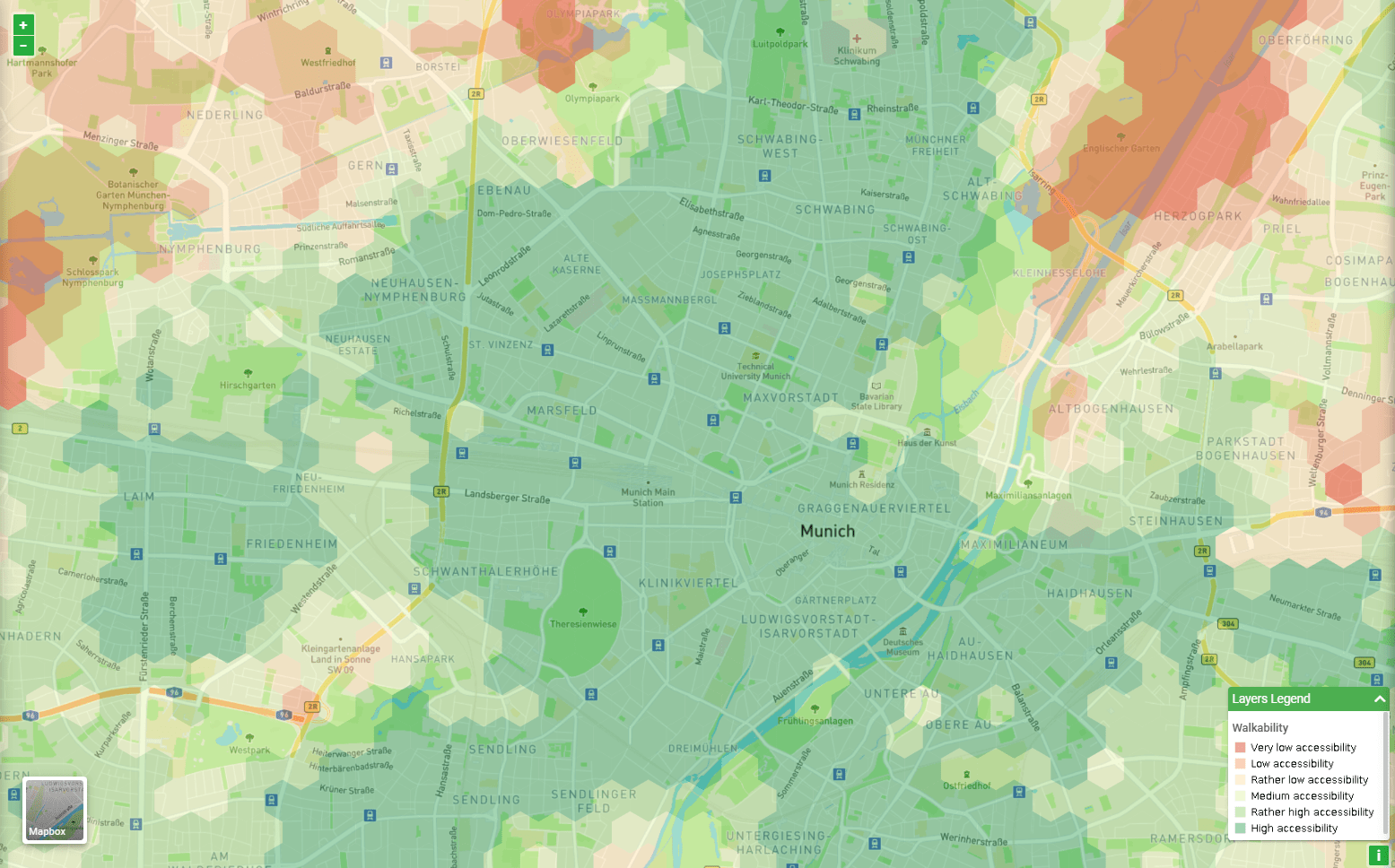#### 1. Calculation

The calculation of the heatmap is calculated with the help of gravity-based measures and can be operationalized as: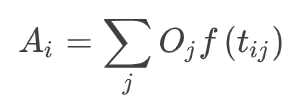where the accessibility A of origin i is the sum of all opportunities O available at destinations j weighted by some function of the travel time tij between i and j. GOAT uses the modified gaussian function as an impedance function for the calculation: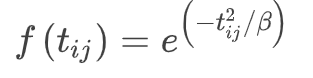Travel times are computed in seconds. As cut-off value 15 minutes is used for the mode walking, this means that destination that are further away then 15 minutes are not considered in the calculation of the index. The sensitivity parameter defines how accessibility changes with increasing travel time. As the sensitivity parameter is decisive when measuring accessibility, GOAT allows you to adjust them. The following graphs show the influence of the sensitivity parameter on accessibility.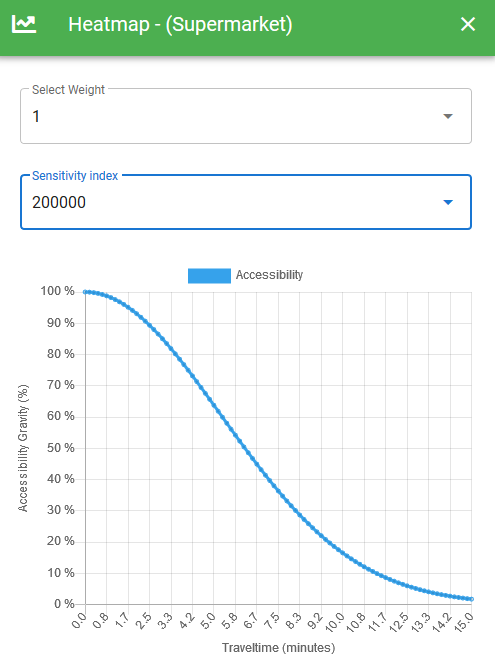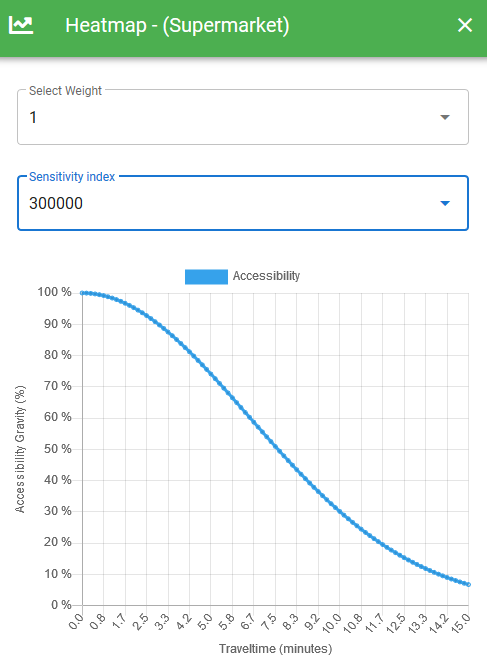#### 2. Classification

In order to classify the accessibility levels that were computed for each grid cell, a classification based on quintiles is used.

#### 3. Example of calculation

##### 3.1 Calculation travel times

The following example illustrates how the gravity-based heatmap is computed. The travel times are calculated for each grid cell to the concerning destination on the street network.For one grid cell the calculation could be done as in the following examples:

Uniform sensitivity parameter: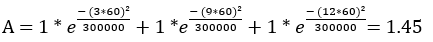Varying sensitivity parameter for Hypermarket:##### 3.2 Calculation with uniform sensitivity parameter

In the first case we want to calculate the accessibility to groceries in 15min (β=300000). This means the sensitivity parameter is the same for every category of grocery.##### 3.3 Calculation with different sensitivity indices

In the second case we calculate the accessibility to groceries in 15min (β=300000 and β=400000). This means the sensitivity parameter depends on the categories of grocery. For this example, we used β= 400000 for the type of grocery hypermarket and β= 300000 for discount supermarket and supermarket.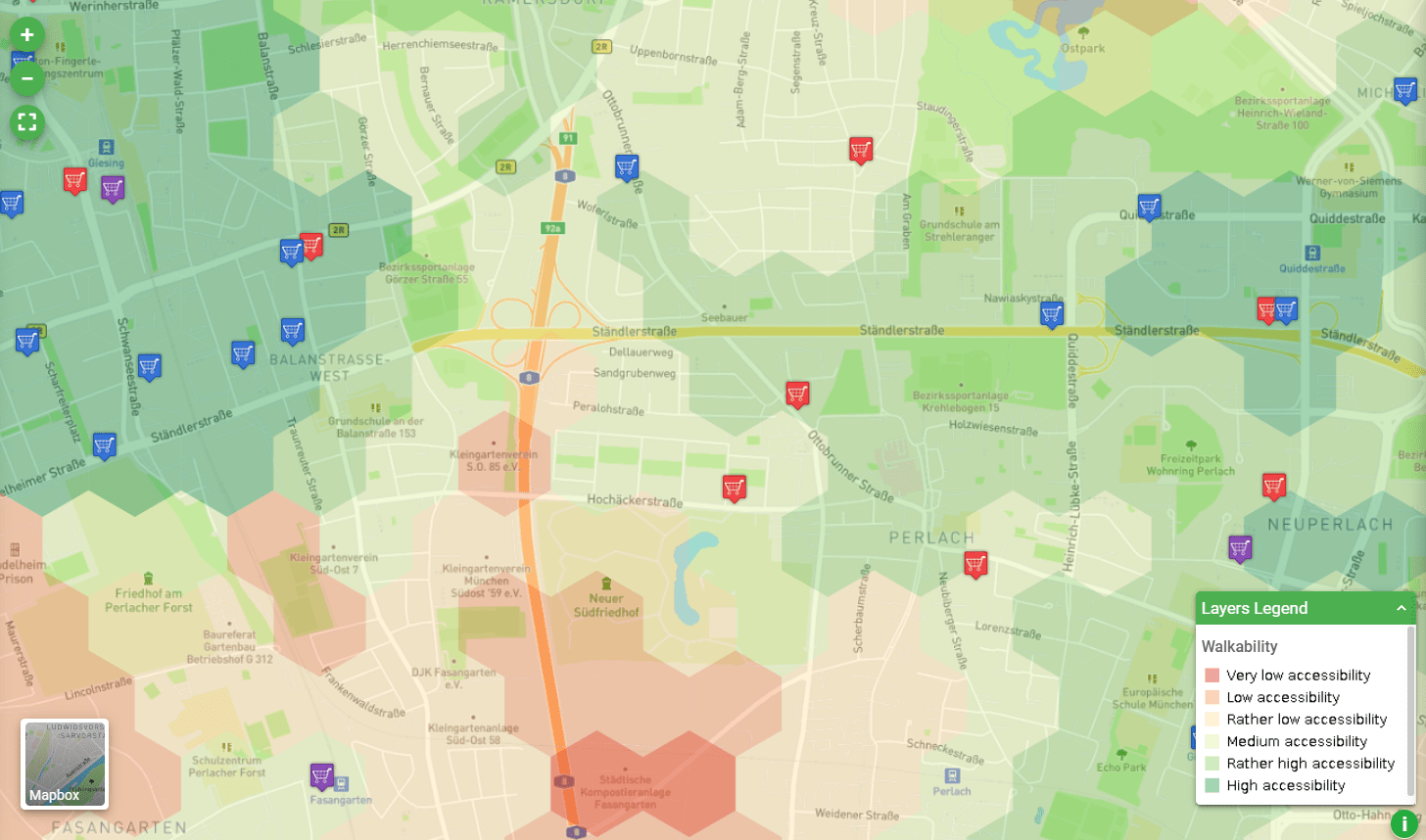If both examples are compared significant changes in accessibility can be observed, as in the second example the sensitivity parameter is chosen in favor of hypermarkets.

Kwan, Mei-Po. 1998. “Space-Time and Integral Measures of Individual Accessibility: A Comparative Analysis Using a Point-Based Framework.” Geographical Analysis 30 (3): 191–216. https://doi.org/10.1111/j.1538-4632.1998.tb00396.x.

Vale, D.S., and M. Pereira. 2017. “The Influence of the Impedance Function on Gravity-Based Pedestrian Accessibility Measures: A Comparative Analysis.” Environment and Planning B: Urban Analytics and City Science 44 (4): 740–63. https://doi.org/10.1177%2F0265813516641685.

Higgins, Christopher D. 2019. “Accessibility Toolbox for R and ArcGIS.” Transport Findings, May. https://doi.org/10.32866/8416.